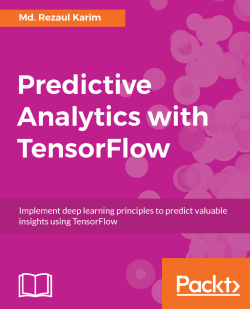# Predictive Analytics with TensorFlow4.8 (8 reviews total)
By Md. Rezaul Karim

• Constantly updated with 100+ new titles each month
• Breadth and depth in over 1,000+ technologies

Predictive analytics discovers hidden patterns from structured and unstructured data for automated decision-making in business intelligence.

This book will help you build, tune, and deploy predictive models with TensorFlow in three main sections. The first section covers linear algebra, statistics, and probability theory for predictive modeling.

The second section covers developing predictive models via supervised (classification and regression) and unsupervised (clustering) algorithms. It then explains how to develop predictive models for NLP and covers reinforcement learning algorithms. Lastly, this section covers developing a factorization machines-based recommendation system.

The third section covers deep learning architectures for advanced predictive analytics, including deep neural networks and recurrent neural networks for high-dimensional and sequence data. Finally, convolutional neural networks are used for predictive modeling for emotion recognition, image classification, and sentiment analysis.

Publication date:
November 2017
Publisher
Packt
Pages
522
ISBN
9781788398923

## Chapter 1. Basic Python and Linear Algebra for Predictive Analytics

Predictive analytics (PA) is the use of data, statistical algorithms, and machine learning techniques to identify the likelihood of future outcomes based on historical data. The goal is to go beyond knowing what has happened to provide the best assessment of what will happen in the future. However, before we start developing predictive analytics models, knowing basic linear algebra, statistics, probability, and information theory with Python is a mandate. We will start with the basic concepts of linear algebra with Python.

In a nutshell, the following topics will be covered in this chapter:

• What are predictive analytics and why do we use them?

• What is linear algebra?

• Installing and getting started with Python

• Vectors, matrices, and tensors

• Linear dependence and span

• Principal component analysis (PCA)

• Singular value decomposition (SVD)

• Predictive modeling tools in Python

## A basic introduction to predictive analytics

We will refer to a famous definition of machine learning by Tom Mitchell, where he explained what learning really means from a computer science perspective:

"A computer program is said to learn from experience E with respect to some class of tasks T and performance measure P, if its performance at tasks in T, as measured by P, improves with experience E"

Based on this definition, we can conclude that a computer program or machine can:

• Learn from data and histories

• Can be improved with experience

• Interactively enhance a model that can be used to predict an outcome

Typical machine learning tasks are concept learning, predictive modeling, clustering, and finding useful patterns. The ultimate goal is to improve the learning in such a way that it becomes automatic: so that no human interactions are needed anymore or reduce the level of human interaction as much as possible.

Predictive analytics on the other hand is the process of extracting useful information from historical facts, and stream data (consisting of live data objects) in order to determine hidden patterns and predict future outcomes and trends.

### Note

What doesn't predictive analytics do?

Predictive analytics does not tell you what will happen in the future, rather it is about creating predictive models that place a numerical value, or score, on the likelihood of a particular event to happen in the future with an acceptable level of reliability, and includes what-if scenarios and risk assessment.

### Why predictive analytics?

In the area of business intelligence, with the right operations management platform, decision-makers are capable of managing all of the business-related inputs, events, and data that provide real-time insight to the enterprise level. Subsequently, predictive models can be used to identify useful patterns from historical, transactional, and recent data to identify potential risks and opportunities. Therefore, it is gaining much attention and wide acceptance. Furthermore, using the traditional reporting and monitoring tools, you have the ability to move from the reactive operations to proactive operations. PA helps move beyond this to plan for the future and identify new areas of business for profit and productivity.

### Working principles of a predictive model

Being at the core of predictive analytics, many machine learning functions can be formulated as a convex optimization problem for finding a minimizer of a convex function f that depends on a variable vector w (weights), which has d records. Formally, we can write this as the optimization problem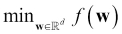, where the objective function is of the form: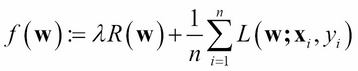Here the vectors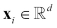are the training data points for 1in, and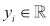are their corresponding labels that we want to predict eventually. We call the method linear if L(w;x,y) can be expressed as a function of wTx and y.

The objective function f has two components: i) a regularizer that controls the complexity of the model, and ii) the loss that measures the error of the model on the training data. The loss function L(w;) is typically a convex function in w. The fixed regularization parameter λ≥0 defines the trade-off between the two goals of minimizing the loss on the training error and minimizing model complexity to avoid overfitting. For more detailed discussion, interested readers should refer to Chapter 7, Using Deep Neural Networks for Predictive Analytics.

A more simplified understanding can be gained from figure 1: you have the current data or observations. Now it's your shot to use the black box to predict the future outcome based on the current data and historical facts. In this context, all the undecided values are called parameters, and the description–that is, the black box, is a PA model: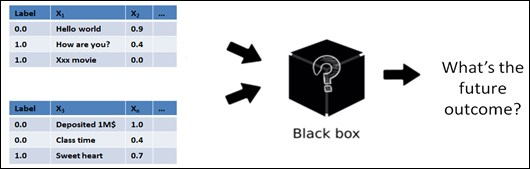Figure 1: the main task in predictive analytics is predictive modeling–that is, using the black box

As an engineer or a developer, you have to write an algorithm that will observe existing parameters/data/samples/examples to train the black box and figure out how to tune parameters to achieve the best model for making predictions before the deployment. Wow, that's a mouthful! Don't worry; this concept will be clearer in upcoming chapters.

In machine learning, we observe an algorithm's performance in two stages: learning and inference. The ultimate target of the learning stage is to prepare and describe the available data, also called feature vector, which is used to train the model.

The learning stage is one of the most important stages, but it is also truly time-consuming. It involves preparing a list of vectors also called feature vectors (most of the time) from the training data after transformation so that we can feed them to the learning algorithms. On the other hand, training data also sometimes contains impure information that needs some pre-processing such as cleaning.

Once we have the feature vectors, the next step in this stage is preparing (or writing/reusing) the learning algorithm. The next important step is training the algorithm to prepare the predictive model. Typically, (and of course based on data size), running an algorithm may take hours (or even days) so that the features converge into a useful model as shown in the following figure: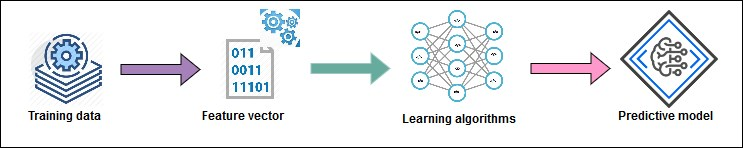Figure 2: Learning and training a predictive model – it shows how to generate the feature vectors from the training data to train the learning algorithm that produces a predictive model

### Note

Common predictive analytics methods

Common predictive analytics methods include regression analysis, classification, time series forecasting, association rule mining, clustering, recommendation systems and text mining, sentiment analysis, and much more. Now to prepare the feature vectors, we need to know a little bit about mathematics, statistics, and so on.

The second most important stage is the inference that is used for making an intelligent use of the model such as predicting from the never-before-seen data, making recommendations, deducing future rules, and so on. Typically, it takes less time compared to the learning stage and sometimes even in real time, as shown in the following figure: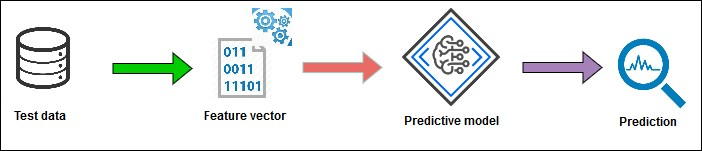Figure 3: Inferencing from an existing model towards predictive analytics (feature vectors are generated from unknown data for making predictions)

Thus, inferencing (see figure 4 for more) is all about testing the model against new (that is, unobserved) data and evaluating the performance of the model itself. However, in the whole process and for making the predictive model a successful one, data acts as the first-class citizen in all machine learning tasks.

In reality, the data that we feed to our machine learning systems must be mathematical objects, such as vectors, matrices, or graphs (in later chapters, we will refer to them as tensors to make it clearer) so that they can consume such data: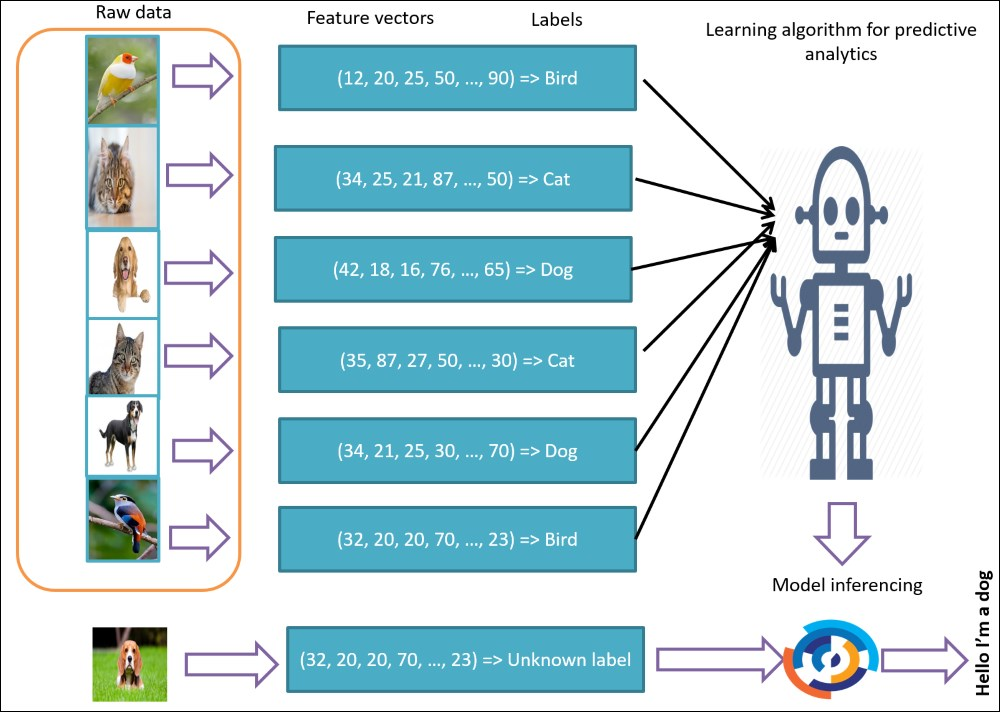Figure 4: Feature vectors are everywhere - they are used in both learning and inferencing stages in predictive analytics

Depending on the available data and feature types, the performance of your predictive model can vacillate dramatically. Therefore, selecting the right features is one of the most important steps before the inferencing takes place. This is called feature engineering, which can be defined as follows:

### Note

Feature engineering

In this process, domain knowledge about the data is used to create only selective or useful features that help prepare the feature vectors to be used so that a machine learning algorithm works.

For example, buying a car; you often see features such as model name, color, horse-power, price, and a number of seats. Thus considering these features, buying a car is not a trivial problem. The general machine learning rule of thumb is that the more data there is, the better the predictive model. However, having more features often creates a mess so the performance degrades drastically: especially if the dataset is high-dimensional and this phenomenon is called the curse of dimensionality. We will see some examples in following sections.

In addition, we also need to know how to represent and use such objects through better representation and transformation. These include some basic (and sometimes advanced maths), statistics, probability, and information theory.

For now, this is enough learning. Let's focus on learning some non-trivial topics of linear algebra that could cover vectors, matrix, graphs, and so on. In Chapter 2, Statistics, Probability and Information Theory for Predictive Modeling, we will learn the basic statistics, probability, and information theory needed for developing PA models. These will be your helping hand as well as basic building blocks for the TensorFlow-based PA throughout subsequent chapters.

## A bit of linear algebra

Linear algebra is a branch of pure mathematics that deals with linear sets of equations and their transformation properties such as the analysis of rotations in space, least squares fitting (LSF), solving linear and differential equations, matrix operation, determination of a circle passing through given points in a vector space over a field, and so on.

You might have heard about the linear regression, which is an example of solving a linear equation where data is represented in the form of linear equations: y = Ax. However, in contrast to classical algebra, linear algebra often deals with matrices and vectors. In practice, more complex operations are used in data representation and model building–that is, in a learning algorithm using the notation and formalisms from linear algebra.

### Programming linear algebra

As a developer, data scientist, or engineer, you may wish to clutch a programming environment and start coding up vectors, matrix multiplication, PCA, SVD, and QR decompositions with test data. The following are some widely used options that you might like to consider and explore:

• SciPy:A Python-based ecosystem for open-source software for mathematics, science, and engineering. This is very easy and is lots of fun if you are a Python programmer with clean syntax.

• Linear algebra package (LAPACK): is a successor to LINPACK and a standard software library for numerical linear algebra (NLA). It offers numerous routines for solving systems such as linear equations, linear least squares, eigenvalue, eigenvector, singular value decomposition, matrix factorizations, and Schur decomposition.

• Basic linear algebra subprograms (BLAS): offers numerous routines as the standard building blocks for performing basic vector and matrix operations.

• NumPy: is the fundamental package for scientific computing in Python. It has a very powerful N-dimensional array object for a multi-dimensional container of generic data and numerical operation and broadcasting functions.

• Pandas: next to SciPy, BLAS, LAPACK, and NumPy, pandas is one of the most widely used Python libraries for data science. It has some expressive data structures straight away!

Well, enough has been said about linear algebra. Now it's time to discuss how to prepare our development environment for learning LA before getting started with Python and TensorFlow for the predictive analytics in upcoming chapters. From my personal experience, Python is a good candidate for learning and implementing LA. Thus, let's have a quick look at how to install and configure Python on different platforms.

## Installing and getting started with Python

Python is one of the most popular programming languages. It is a high-level, interpreted, interactive, and object-oriented scripting language. Unfortunately, there has been a big split between Python versions: 2 versus 3, which could make things a bit confusing to newcomers. You can see the major difference between them at https://wiki.python.org/moin/Python2orPython3. But don't worry; I will lead you in the right direction for installing both major versions.

### Installing on Windows

On the Python download page at https://www.python.org/downloads/, you'll find the latest release of Python 2 or Python 3 (2.7.13 and 3.6.1, respectively, at the time of writing). You can now select and download the installer (`.exe`) of either version. Installation is similar to installing other software on Windows.

Let's assume that you have installed both versions and now it's time to add the installation path to the environmental variables.

For doing so click on Start, and then type `advanced system settings`, then select the View advanced system settings | System Properties | Advanced | Environment Variables... button:Figure 5: Creating a system variable for Python

Python 3 is usually listed in the User variables for Jason, but Python 2 is listed under the System variables as follows: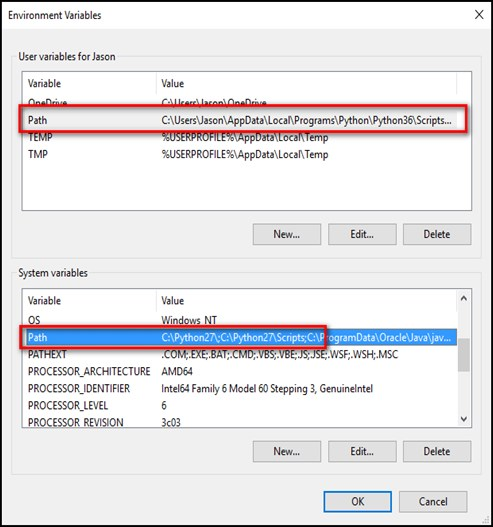Figure 6: Showing how to add the Python installation location as system path

There are a few ways you can remedy this situation. The simplest way is to make changes that can give us access to `python` for Python 2 and `python3` for Python 3. For this, go to the folder where you have installed Python 3. It should be something like this: `C:\Users\[username]\AppData\Local\Programs\Python\Python36` by default.

Make a copy of the `python.exe` file, and rename that copy (not the original) to `python3.exe` as shown in the following screenshot: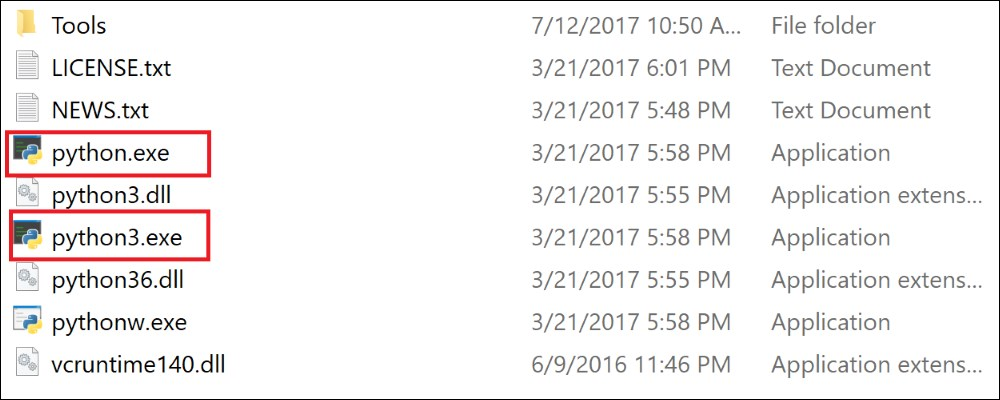Figure 7: Fixing Python 2 versus Python 3 issue

Open a new Command Prompt (the environmental variables refresh with each new Command Prompt you open), and type `python3 --version`: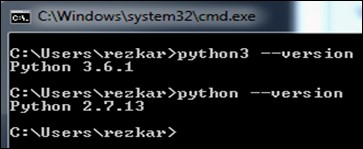Figure 8: Showing Python 2 and Python 3 version

Fantastic, now you're ready for whatever Python project you want to tackle.

### Installing Python on Linux

For those of you who are new to Python, Python 2.7.x and 3.x are automatically installed on Ubuntu. Make sure to check if `Python 2` or `Python 3` is installed using the following command:

```\$ python -V
>> Python 2.7.13
\$ which python
>> /usr/bin/python
```

For Python 3.3+ use the following:

```\$ python3 -V
>> Python 3.6.1
```

If you want a very specific version:

```\$ sudo apt-cache show python3
\$ sudo apt-get install python3=3.6.1*
```

### Installing and upgrading PIP (or PIP3)

The `pip` or `pip3` package manager usually comes with your Ubuntu. Make check to sure if `pip` or `pip3` is installed using the following command:

```\$ pip -V >> pip 9.0.1 from /usr/local/lib/python2.7/dist-packages/pip-9.0.1-py2.7.egg (python 2.7)
```

For Python 3.3+ use the following:

```\$ pip3 -V >> pip 1.5.4 from /usr/lib/python3/dist-packages (python 3.4)
```

It is to be noted that `pip` version 8.1+ or `pip3` version 1.5+ are strongly expected to give better results and smooth computation. If version 8.1+ for `pip` and 1.5+ for `pip3` are not installed, see the following command to either install or upgrade to the latest `pip` version:

```\$ sudo apt-get install python-pip python-dev
```

For Python 3.3+, use the following command:

```\$ sudo apt-get install python3-pip python-dev
```

### Installing Python on Mac OS

Before installing the Python, you should install a C compiler. The fastest way of doing so is to install the `Xcode` command-line tools by running the following command:

```xcode-select –install
```

Alternatively, you can also download the full version of `Xcode` from the Mac App Store.

If you already have `Xcode` installed on your Mac machine, do not install OSX-GCC-Installer. In combination, you can experience some unwanted issues that are really difficult to diagnose and get rid of.

Although Mac OS comes with a large number of Unix utilities, however, one key component called Homebrew is missing, which can be installed using the following command:

```\$ /usr/bin/ruby -e "\$(curl -fsSL https://raw.githubusercontent.com/Homebrew/install/master/install)"
```

Set the Homebrew installation path to the `PATH` environment variable to the `~/.profile` file by issuing the following command:

```export PATH=/usr/local/bin:/usr/local/sbin:\$PATH
```

Now, you're ready to install Python 2.7.x or 3.x. For Python 2.7.x issue the following command:

```\$ brew install python
```

For Python 3 issue the following command:

```\$ brew install python3
```

### Installing packages in Python

Additional packages (other than built-in packages) that will be used throughout this book can be installed via the `pip` installer program. We have already installed Python `pip` for Python 2.7.x and Python 3.x. Now to install a Python package or module, you can execute `pip` on the command line (Windows) or terminal (Linux/Mac OS):

```\$ sudo pip install PackageName # For Python3 use pip3
```

However, already installed packages can be updated via the `--upgrade` flag by issuing the following command:

```\$ sudo pip install PackageName –upgrade # For Python3, use pip3
```

## Getting started with Python

In this sub-section, we will see some examples on how to get familiar with Python programming. I assume you already know the basic Python. However, I will provide some basic things in Python that will be needed in upcoming sections and chapters.

### Python data types

Python has five standard data types as follows:

• Numbers

• String

• List

• Tuple

• Dictionary

Besides these, Python supports four different numerical types, such as:

• `int` (signed integers)

• `long` (long integers, can be represented in octal and hexadecimal too)

• `float` (floating point real values)

• `complex` (complex numbers)

Now you can assign values to the variables using the `=` sign as follows:

```>>> counter = 100.50        # A floating point
>>> age   = 32              # An integer assignment
>>> name    = "Reza"        # A string
```

Python also allows you to assign a single value to several variables concurrently. For example:

```>>> x = y = z = 50
```

Here we have created an integer with the value `50` and subsequently, all three variables are assigned to the same memory location. Furthermore, you can also assign multiple objects to multiple variables with ease.

For example:

```>>> x,y,z = 50,30,"Reza"
```

Two integer objects (that is, `50` and `30`) will assign to variables `x` and `y` respectively. On the other hand, variable `z` will be assigned to string `Reza`.

### Using strings in Python

Strings in Python are identified as a contiguous set of characters represented in quotation marks. Indexes start at `0` in the beginning of the string. The `+` sign is used as the string concatenation operator. Whereas the `*` is the repetition operator.

For example:

```>>> message = 'Hello, world!'
>>> print message #complete string will be printed
>>> print message #Only the first character will be printed
>>> print message[2:5] #Characters from 3rd to 5th will be printed
>>> print message * 2      # Prints string two times
>>> print message + "TEST" # Prints concatenated string
```

The preceding lines should produce the following output:

`Hello, world!`

`H`

`llo`

`Hello, world!Hello, world!`

`Hello, world!TEST`

### Using lists in Python

Lists are one of the most versatile objects used in Python. A list contains items separated by commas enclosed within square brackets–that is, `[]`. Values in a list can be accessed using the slice operator (`[ ]` and `[:]`) with indexes starting at `0` in the beginning and the end at `n-1` considering the length of the list is n. The concatenation and repetition operation is similar to strings in Python. Let's see some examples:

```>>> list1 = [ 'Ireland', 1985 , 4.5, 'John Rambo']
>>> list2 = ['USA', 1982 , 6.5, 'Sylvester Stallone']
>>> print list1     # Prints the complete list
>>> print list1    # Prints only the first element of the list
>>> print list1[1:3]  # Prints elements starting from 2nd to 3rd
>>> print list1[2:]   # Prints elements starting from 3rd element
>>> print list1 * 2   # Prints the list 2 times
>>> print list1 + list2 # Prints the concatenated lists
```

This produces the following output:

` ['Ireland', 1985, 4.5, 'John Rambo'] `

` Ireland`

` [1985, 4.5]`

` [4.5, 'John Rambo']`

` ['Ireland', 1985, 4.5, 'John Rambo', 'Ireland', 1985, 4.5, 'John Rambo']`

` ['Ireland', 1985, 4.5, 'John Rambo', 'USA', 1982, 6.5, 'Sylvester Stallone']`

### Using tuples in Python

A tuple is another sequence data type similar to the list consisting of values separated by commas, but enclosed within parentheses. While the elements and size in a list can be changed, a tuple cannot be updated. Thus you can think of a tuple as a read-only list:

```>>> tuple1 = ('Ireland', 1985, 4.5, 'John Rambo')
>>> tuple2 = ('USA', 1982, 6.5, 'Sylvester Stallone')
>>> print tuple1     # Prints the complete list
>>> print tuple1  # Prints only the first element of the list
>>> print tuple1[1:3] # Prints elements starting from 2nd to 3rd
>>> print tuple1[2:]  # Prints elements starting from 3rd element
>>> print tuple1 * 2   # Prints the list 2 times
>>> print tuple1 + tuple2 # Prints the concatenated lists
```

This produces the following output:

`>>> ('Ireland', 1985, 4.5, 'John Rambo') `

` Ireland`

` (1985, 4.5]`

` (4.5, 'John Rambo')`

` ('Ireland', 1985, 4.5, 'John Rambo', 'Ireland', 1985, 4.5, 'John Rambo')`

` ('Ireland', 1985, 4.5, 'John Rambo', 'USA', 1982, 6.5, 'Sylvester Stallone') `

### Using dictionary in Python

Dictionaries are a kind of hash table. You can compare them with associative arrays or hashes in Perl. A dictionary consists of key-value pairs: where a key can be any Python type, but mostly are numbers and strings. A value on the other hand, can also be any arbitrary Python object. In Python, a dictionary is enclosed by curly braces (`{}`). The values are usually assigned and can be accessed using square braces (`[]`) or using the `get()` method. For example:

• An empty dictionary:

```>>> mydict = {}
```
• A dictionary with integer keys and string values:

```>>> mydict = {1: 'apple', 2: 'ball', 3: 'cat'}
```
• A dictionary with mixed keys:

```>>> mydict = {'name': 'John Rambo', 'numbers': [2, 4, 3]}
```
• Printing the whole dictionary:

```>>> print(mydict)
```

Output:` {'name': 'John Rambo', 'numbers': [2, 4, 3]} `

• Accessing dictionary element using `[]`:

```>>> print(mydict['name'])
```

Output: `John Rambo`

• Accessing dictionary element using the `get()` method:

```>>> print(mydict.get('numbers'))
```

Output: `[2, 4, 3]`

• Updating a value:

```>>> mydict['name'] = 'Asif Karim'
>>> print(mydict)
```

Output: `{'name': 'Asif Karim', 'numbers': [2, 4, 3]}`

```>>> mydict['address'] = 'Aachen, Germany'
>>> print(mydict)
```

Output: `{'address': 'Aachen, Germany', 'name': 'Asif Karim', 'numbers': [2, 4, 3]}`

• Removing an arbitrary item:

```>>> mydict.popitem()
>>> print(mydict)
```

Output: `{'name': 'Asif Karim', 'numbers': [2, 4, 3]}`

• Removing all items:

```>>> mydict.clear()
>>> print(mydict)
```

Output: `{}`

### Using sets in Python

A set can be created by placing any number of items inside curly braces `{}`, separated by a comma. Items in a set can be of different types (integer, float, tuple, string, and so on). Alternatively, a set can be created using the built-in function `set()` of Python. For example:

• A set of integers:

```>>>> mySet = {1, 2, 3, 4, 5}
>>> print(mySet)
```

Output: `set([1, 2, 3, 4, 5])`

• A set of mixed datatypes:

```>>> mySet = {4.0, "John R ambo", (1, 2, 3, 4, 5), 9}
>>> print(mySet)
```

Output: `set([9, (1, 2, 3, 4, 5), 4.0, 'John R ambo'])`

• Inserting a single item to existing set:

```>>> mySet.add(2.5)
>>> print(mySet)
```

Output: `set([2.5, 9, (1, 2, 3, 4, 5), 4.0, 'John R ambo'])`

```>>> mySet.update([7,8,9])
>>> print(my_set)
```

Output: `set([2.5, 4.0, 7, 8, 9, (1, 2, 3, 4, 5), 'John R ambo'])`

A particular item can be removed from set using `discard()` and `remove()`:

```>>> mySet.remove(8)
>>> print(mySet)
```

Output: `set([2.5, 4.0, 7, 9, (1, 2, 3, 4, 5), 'John R ambo'])`

```>>> mySet.discard(7)
>>> print(mySet)
```

Output: `set([2.5, 4.0, 9, (1, 2, 3, 4, 5), 'John R ambo'])`

### Note

Set and mutable elements: beware that a set cannot have mutable elements as its member such, list, set, or dictionary.

### Functions in Python

In Python, a function is a first-class citizen: consisting of a group of related statements for performing a specific task. Functions help you gain modularity in your code. Since your program grows larger and larger, functions make it more organized and manageable. Thus it also helps us avoid repetition towards making the code reusable.

The basic syntax of declaring a function in Python is as follows:

```def function_name(parameters):
... statement(s)
return [expression_list]

def absolute_value(x):
if x >= 0:
return x
else:
return -x```

Now the preceding function can be called as follows:

```>>> absolute_value(10)
```

#Output: `10`

```>>> absolute_value(-10)
```

#Output: `10`

### Note

Lines and indentation in Python: be aware that Python does not provide any brackets/braces (such as Java, C++, and so on.) to indicate blocks of code for a method or class definitions or flow control. Rather blocks of code are denoted by a line indentation. Fortunately or unfortunately, this convention is strictly enforced. The number of spaces in the indentation is variable. However, it is known that all statements within the block must be indented the same amount.

Now it's time to discuss some Object Oriented Programming (OOP) concepts. Like other OOP, classes in Python are also basic building blocks. However, for simplicity, we are not going to discuss most of the OOP concepts in this chapter but readers will get to know them in upcoming chapters.

### Classes in Python

Similar to functions, a class can be defined using the keyword class. Once you create a class in Python, it creates a new local namespace; where all the attributes are defined. Well, an attribute can be data, set, list, dictionary, array, or a function:

```class MyAbsClass:
number = 20
name = "John Rambo"
def __init__(self, number=10):
self.real = number
def absolute_value( x):
if x >= 0:      return x
else:
return -x```

Now if we want to access the properties of the preceding class, we have to create an object of that class. This is also called instantiation of that class. Creating an object is similar to a function call:

```>>> obj = MyAbsClass()
```

### Note

Instantiation

An object is used to call an instance of a class. This process is called instantiation.

Let's see the whole class containing some data and methods as follows:

```class MyAbsClass:
number = 20
name = "John Rambo"
def __init__(self, number=10):
self.real = number
def absolute_value( x):
if x >= 0:
return x
else:
return -x
obj = MyAbsClass()
value = obj.absolute_value(-10)
print("The absolute value of -10 is : "+ str(value))
print(obj.number)
print(obj.name)```

Output:

`The absolute value of -10 is: 10`

`20`

`John Rambo `

Now let's move to the next section, where we will discuss vectors, matrix, graph and tensors, and so on. Interested readers can refer to this URL for more extensive materials: https://www.programiz.com/python-programming/.

## Vectors, matrices, and graphs

Learning how to perform several operations on matrices including inverse, eigenvalues, and determinants are some fundamental things before using some advanced topics such as (PCA, SVD, and so on. Thus, in this section, we will discuss vectors, metrics, and tensors, which are some fundamental topics for learning predictive analytics.

### Vectors

The vector object is not a displayable object but is a powerful aid to 3D computations. Its properties are similar to vectors used in science and engineering. It can be used together with NumPy arrays.

For example, suppose an airplane is flying due north, there's a wind coming however from the north-west (see below figure). Now the question is how will the plane survive and move to the north?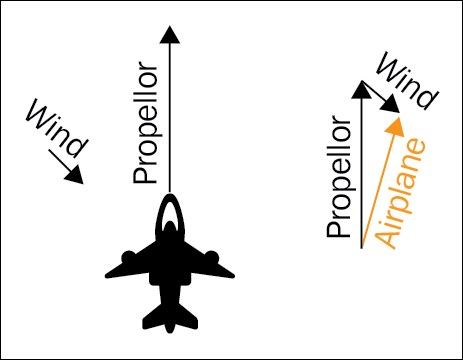If you look at the preceding figure carefully, there are two types of velocity that are active. The velocity caused by the wind and the propeller, respectively. The resultant velocity results in a marginally slower ground speed legend the plan east of north. Now the thing is if you observe the plane from the ground, it would seem that the plane is being moved sideways slightly as shown in the following figure: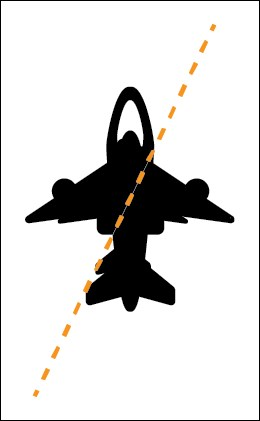Alternatively, you might have seen the birds struggling against the strong winds that seem to fly sideways. Using a vector of linear algebra, we can have a better explanation as to why that happens.

Python provides several modules for computing vector operations. For example, vectors is such a module that can be used to return a vector object with the given components, which are made to be floating-point (that is, 3 is converted to 3.0). Vectors can be added or subtracted from each other, or multiplied by an ordinary number. For example:

```import numpy as np
from vectors import Point, Vector
v1 = Vector(1, 2, 3)
v2 = Vector(10, 20, 30)```

### Note

Be aware that the Vectors module used for the preceding example code does not have support for Python3. So to install this module in Python 2, issue the following command on Linux:

```\$ pip install vectors
```

We can add a real number to a vector or compute the vector sum of two vectors as follows:

```print(v1.add(10))
>> Vector(11.0, 12.0, 13.0)
print(v1.sum(v2))
>> Vector(11.0, 22.0, 33.0)
```

In the preceding cases, both methods return a vector instance. We can get the magnitude of the vector easily:

```Print(v1.magnitude())
>> 3.7416573867739413
```

We can multiply a vector by a real number. The following returns a vector instance:

```print(v1.multiply(4))
>> Vector(4.0, 8.0, 12.0)
```

To find the dot product of two vectors:

```print v1.dot(v2)
>> 140.0
```

To use angle theta on the dot function, check the following case for which the dot product returns a real number:

```print(v1.dot(v2, 180))
>> -4800.49306298
```

To perform the cross product of two vectors which returns a vector instance that is always perpendicular (90 degrees) to the other two vectors:

```v1.cross(v2)
>> Vector(0, 0, 0)
```

We can also find the angle theta between two vectors. It is to be noted that the angle is measured in degrees:

```v1.angle(v2)
>> 0.0
```

It is also possible to check if the two given vectors are parallel, perpendicular, or non-parallel to each other. For the following cases the result is either true or false:

```v1.parallel(v2)
>> True
v1.perpendicular(v2)
>> False
```

### Note

For the mathematical explanation, please refer to this URL to get more insight: https://www.mathsisfun.com/algebra/vectors.html.

In the previous section, we mentioned buying a car that has some resemblance to feature engineering. Now let's see an example of how vectors could help us to select appropriate features.

Suppose you have the feature vectors of some potential cars. Now it's possible to figure out which two cars are similar by defining a distance function out of the feature vectors. One thing should be remembered: that comparing similarities and dissimilarities between data objects are one of the fundamental components in predictive analytics. Linear algebra helps us represent objects towards comparing.

One of the standard ways of doing so is calculating the Euclidian distance is an intuitive thinking of points in space. Suppose you have the following two feature vectors X = (X1, X2… Xn) and Y= (Y1, Y2… Yn). Now the Euclidian distance can be calculated as follows: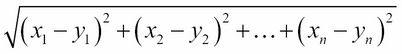Thus if you have two points, for example (0, 2), (4, 0), the Euclidian distance would be: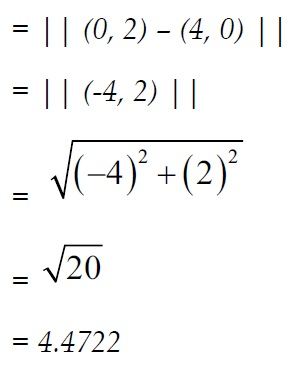This is called L2 norm and it is actually one of the many possible distance functions. In the real world, a more complex distance function is used. We will see it in upcoming chapters.

### Matrices

A matrix is a 2D array for storing real or complex numbers. In a real matrix, all of its elements r belong to R. Similarly, a complex matrix has entries c in C.

Given that two matrices have the same dimension, they can be added together, which results in a new matrix with the same dimensions: each element is the sum of the corresponding elements of the previous matrices. Suppose we have the following matrix `A` and `B` as follows:

```A = np.matrix(
[[3, 2],
[4, 6]])
B = np.matrix(
[[1, 4],
[2, 0]])
```

Now the addition of the precoding matrices can be computed as follows:

```C = A + B
print(C)

>> [[ 8 -5]
[ 6 15]]
```

#### Matrix subtraction

Similar to addition, in matrix subtraction, each element of one matrix is subtracted from the corresponding element of the other. If a scalar is subtracted from a matrix, the former is subtracted from every element of the latter:

```A = np.matrix(
[[1, 4],
[2, 9]])

B = np.matrix(
[[7, -9],
[4, 6]])

>> [[-6 13]
[-2  3]]
```
##### Multiplying two matrices

Finding the product of two matrices is also required in many cases. When two matrices are multiplied, the result is simply expanded with each column of the result obtained by using the corresponding column of the second matrix. Suppose we have the following matrix `A` and `B` as follows:

```A = np.matrix(
[[1, 4],
[2, 0]])
B = np.matrix(
[[3, 2],
[4, 6]])
```

Now the addtion of the preceding matrixes can be computed as follows:

```C = A * B
print(C)
>> [[23 15]
[50 36]]
```

Furthermore, it is also required sometimes to add a constant to each element in a matrix, this is called summing of a matrix and a scalar. Let's add a constant, say `8`, to matrix `A` as follows:

```B =  A + 8
Print(B)
>> [[ 9 12]
[10  8]]
```

#### Finding the determinant of a matrix

The determinant can be computed from the elements of a square matrix. The determinant of a matrix `A` is denoted `det(A)`, `det A`, or `|A|`. Often, determinant is viewed as the scaling factor of the transformation described by the matrix itself. One of the interesting facts is that the determinant of a product of matrices is always equal to the product of determinants:

```A = np.matrix(
[[3, 2],
[4, 6]])
deter = np.linalg.det(A)
print(deter)
>> 10.0
```

### Finding the transpose of a matrix

In matrix transpose, rows become columns and columns should be rows. Suppose we have the following matrix:

```matrix = np.matrix(
[[3, 6, 7],
[2, 7, 9],
[5, 8, 6]])
transpose = np.transpose(matrix)

>> [[3 2 5]
[6 7 8]
[7 9 6]]
Matrix inversion
```

We have seen addition, subtraction, and multiplication of matrixes, however, there is no such division. Fortunately, there is a matrix construct similar to that of division, and it is central to much of the work of the analyst. The key ingredient is the use of the inverse of a matrix.

Let's see an example:

```matrix = np.matrix(
[[3, 6, 7],
[2, 7, 9],
[5, 8, 6]])
inverse = np.linalg.inv(matrix)
print(inverse)
>> [[ 1.2  -0.8  -0.2 ]
[-1.32  0.68  0.52]
[ 0.76 -0.24 -0.36]]
```

It is to be noted that the multiplication of the original matrix and the inverse one always produces a square matrix also called identity matrix. More formally:

```Inv(A) * A = I
```

### Note

Identity matrix

An identity matrix is a square matrix with ones on the diagonal from the upper left to lower right and zeros elsewhere. For example:

```   I = [[1 0 0],
[0 1 0],
[0 0 1]]
```

### Solving simultaneous linear equations

Matrix inversion is often used to solve a set of simultaneous linear equations. For example, how to find the solution of Ax=B: that is the value of x that satisfies this equation. Suppose we have the following matrix `A` and `B` as follows:

```A = np.matrix(
[[1, 4],
[2, 0]])

B = np.matrix(
[[3, 2],
[4, 6]])
```

Now the solution can be computed by calling the solve method as follows:

```X = np.linalg.solve(A, B)
print(X)
>> [[ 2.    3.  ]
[ 0.25 -0.25]]
```

### Eigenvalues and eigenvectors

In the following figure, original matrix `A` acts by extending the vector x without changing its direction. Thus, x is an eigenvector of matrix `A`; whereas the scale factor λ is the eigenvalue corresponding to the eigenvector x: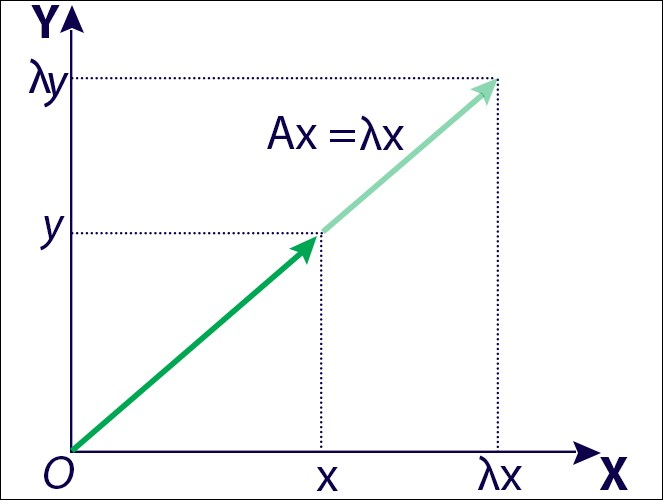Figure 13: demonstrating eigenvalues and eigenvectors

For example:

```matrix = np.matrix(
[[3, 6, 7],
[2, 7, 9],
[5, 8, 6]])
eigvals = np.linalg.eig(matrix)
print(eigvals)
>> (array([ 18.03062661,   0.53948277,  -2.57010939]),
matrix([[-0.52213277, -0.69701957, -0.23035157],
[-0.59400273,  0.64684805, -0.6406727 ],
[-0.6119952 , -0.3094371 ,  0.73244566]]))
```

In the preceding output, the array signifies eigenvalues and the matrix signifies the eigenvector.

## Span and linear independence

The span of vectors v1, v2... vn is the set of linear combinations such that: c1v 1 + c2v2 + ... + cnvn, which is a vector space called V. Now if we further expand this idea such that S = {v1, v2... vn) is a subset of V, then Span(S) is equal to V. More formally, S spans V if and only if every vector v in V can be expressed as a linear combination of vectors in S–that is: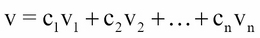Let's see an example, suppose we have the following set S = {(0,1,1), (1,0,1), (1,1,0)}. Obviously, this set spans R3 Therefore, vector (`2`, `4`, `8`) can be expressed as a linear combination of vectors in S.

To solve this, we can say that a vector in R3 has the form v = (x, y, z). Therefore, it would be enough showing that every such v can be expressed as follows:

```(x,y,z) = c1(0, 1, 1) + c2(1, 0, 1) + c3(1, 1, 0)
= (c2 + c3, c1 + c3, c1 + c2)
```

Now the preceding relation can be written more explicitly as follows:

```c2 + c3 = x
c1 + c3 = y
c1 + c2 = z
```

If we can recall our undergraduate mathematics, the preceding relation can be written in matrix form as follows: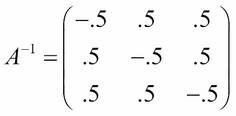Now the preceding relation is pretty expressible in the equation form as follows:

Ax = B

If you look carefully, the determinant of matrix A is 2, that is, det(A) = 2. This also signifies that A is non-singular. Therefore, there exists a solution such that x = A-1B. Now as asking, to write (2, 4, 8) as a linear combination of vectors in S, we now find the following:We also find the following: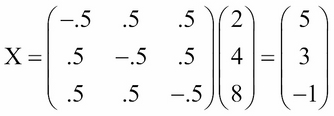Finally, we have:

(2,4,8) = 5(0,1,1) + 3(1,0,1) + (-1)(1,1,0)

So far, we know how to find out if a group of vectors spans over a vector space. Now the question is are there any redundancies in the vectors span? That is, is there a smaller subset of S such that it also Span(S) then one of the given vectors can be rewritten as a linear combination of the others, such that: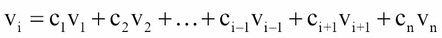If the preceding relation is satisfied then S is a linearly dependent set, otherwise, S is linearly independent. There is another way of checking that a set of vectors are linearly dependent. Now let's see an example of the preceding definition.

Given a set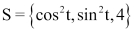. Now it can be seen that S is a linearly dependent set of vectors since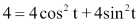Now we know some basic concepts from linear algebra to construct a predictive analytics model, yet often we need to deal with high dimensional data to make the prediction more meaningful by taking out less significant or correlated features. PCA algorithm comes in handy to deal with the curse of dimensionality.

## Principal component analysis

In predictive analytics, most often you will face an issue about the data dimensionality also called the curse of dimensionality. You need to deal with too many variables having less important ones as well. Thus when a dataset has too many variables, there are only a few possible situations you may encounter:

1. You find that most of the variables are correlated–that is, have a mutual relationship or connection, in which one thing affects or depends on another.

2. Then say you lose patience and decided to train the model using the whole data. This results in a very poor accuracy and your boss is unhappy.

3. Naturally, you might be indecisive about what to do next.

4. Finally, you start thinking to get rid of the issue by finding only important variables–that is, feature selection.

Believe it or not, handling this issue is not that difficult, but the usage of some statistical techniques such as factor analysis, singular value decomposition, and principal component analysis help overcome such difficulties.

PCA is a statistical method for extracting important variables from a high-dimensional dataset (having so many variables). In other words, PCA extracts a low-dimensional set. But it tries to capture as much information as possible called components form. This is not full of surprise, but with fewer variables, the interactive visualization becomes more meaningful. In particular, PCA is more useful when dealing with higher dimensional data–that is, at least three dimensions.

### Note

Principal components

In a PCA algorithm, a principal component is a normalized linear combination of the original predictors in a dataset.

The PCA is all about performing operations on a symmetric correlation or covariance matrix. Therefore, the matrix has to be numeric having standardized data. Let's say we have a dataset of dimension 300 (m) × 300 (n). Where m signifies a number of observations and n is the number of predictors–that is, response. Since the dataset is high-dimensional–that is, having n = 300, theoretically there could be n(n-1)/2–that is, 44850 scatter plots for analyzing the available relationship in the variable.

You're right, yes, performing an exploratory analysis on this type of data is really difficult–that is, the curse of dimensionality. One approach could be selecting a subset of n (n << 300) predictors to capture as much information as possible without sacrificing the quality much. Now if you plot such data, the observation in the resultant is a low dimensional space.

For example, the following figure shows the transformation of three-dimensional gene expression data, which is mainly located within a two-dimensional subspace. PCA is then used to visualize this data by reducing the dimensionality of the data. If you look at the graph carefully, you can observe that each subsequent dimension is a linear combination of n features: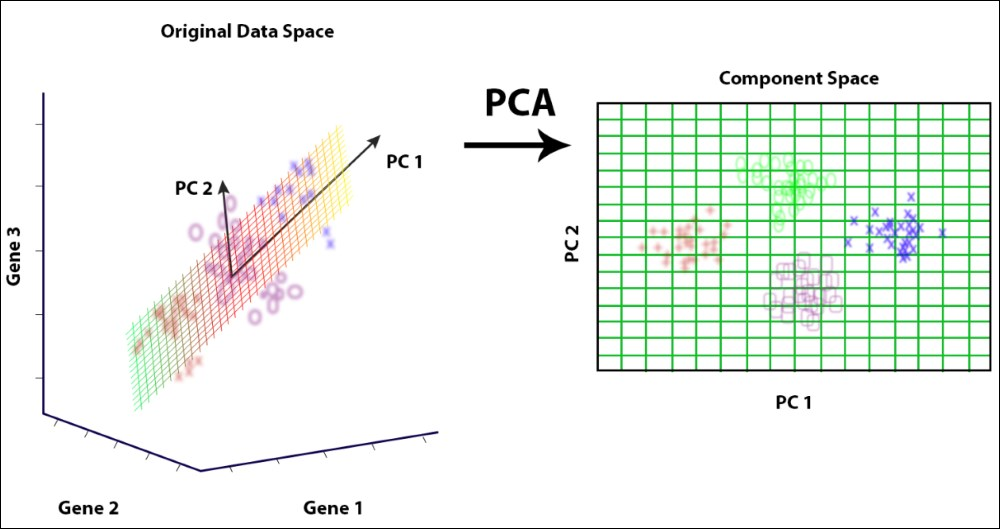Figure 19: Using PCA in bioinformatics with high dimensional data

In the preceding figure, PC1 and PC2 are the principal components.

## Singular value decomposition

If matrix A has a matrix of eigenvectors P that is not invertible, then A does not have Eigen decomposition too. However, if A is an m x n real matrix with m>n, then the original matrix A can be written using a so-called singular value decomposition of the form (as the product of three matrices) U, Σ, V*. Suppose we have the following matrix:

```matrix = np.matrix(
[[6, 8],
[5, 7]] )
```

Now the SVD can be computed by calling the `svd()` method from the NumPy module of Python as follows:

```svd = np.linalg.svd(matrix)
```

This is an array that has three fields–that is, `u`, `sigma`, and `v`:

```U = svd
Sigma = svd
V = svd
```

For better interpretation of the preceding result, let's do some transformation–that is, converting each field as a list as follows:

```U = U.tolist()
Sigma = Sigma.tolist()
V = V.tolist()
```

Let's compute the matrix production consisting of the three components:

```matrix_prod = [[‚\$U\$', ‚', ‚\$\Sigma\$', ‚\$V^*\$', ‚'],
[U, U, Sigma, V, V],
[U, U, Sigma, V, V]]
```

Let's convert the preceding matrix into a table for the SVD:

```table = FF.create_table(matrix_prod)
```

Finally, display the components as follows:

```py.plot(table, filename='Matrix_SVD')
```

The output is as follows: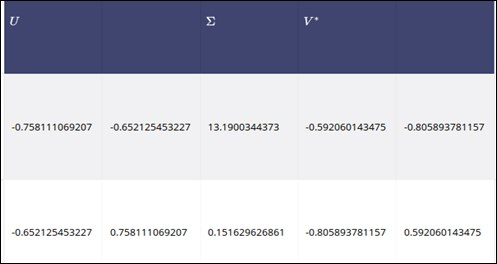Figure 20: Showing each and singular value that are decomposed using SVD

### Data compression in a predictive model using SVD

The SVD is a widely used decomposition technique in computer science, math, and other disciplines. In this section, I will provide a small glimpse of that in a data compression technique. Suppose we have a matrix A with rank 200–that is, the columns of this matrix span a 200-dimensional space. Representing and encoding this large matrix on your PC will take a pretty good amount of memory.

SVD comes at the front end to efficiently handle this issue without sacrificing by approximating the original matrix with one of lower rank. Suppose we approximate it as a matrix with rank 100. Now the question is how close can we get to this matrix by storing only 100 columns? Another question could be that can we use a matrix of rank 20? In other words, is it possible to summarize all of the information of this very dense, (that is, 200-rank) matrix with only a rank 20 matrix?

If we want to keep say 90% of the original information, it would be enough computing of the sums of singular values until we reach 90% of the sum. Consequently, the rest of the singular values will be discarded. Since the SVD algorithm only stores the columns of U and V we greatly reduce the memory usage since we set elements on the diagonal of Σ to 0. Images are represented in a rectangular array where each element corresponds to the grayscale value for that pixel.

For colored images, we have a three-dimensional array of size m x n x 3, where m and n represent the number of pixels vertically and horizontally, respectively, and for each pixel, we store the intensity for colors red, green, and blue. The three signifies that this is a three-dimensional space. Now are going to repeat the preceding low-rank approximation procedure on a larger matrix. The resulting three-dimensional array will be a pretty good approximation of the original image. Here's the original image: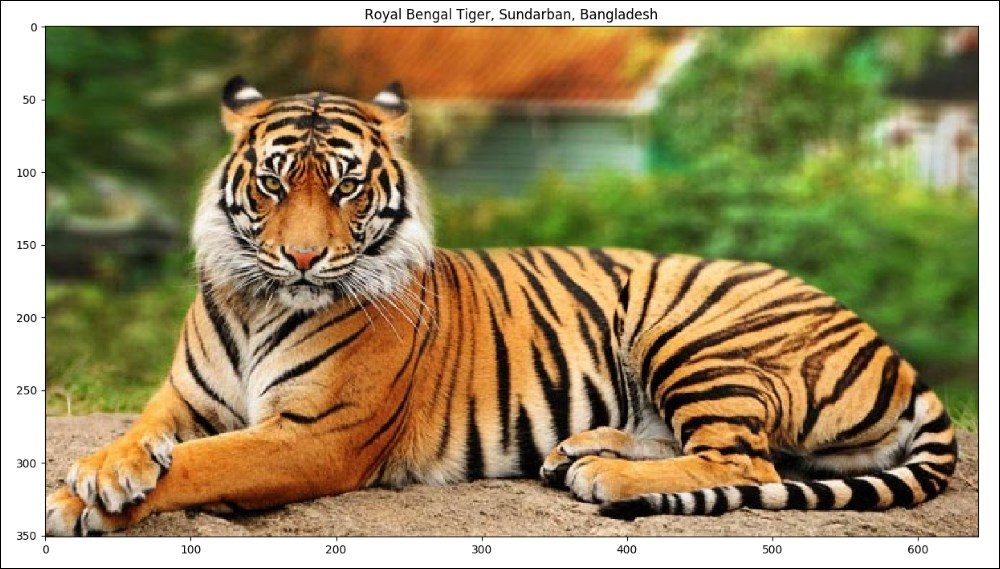Figure 21: Original image having 1000*1600 dimensions and takes 37500 KB

Now at first, we do the singular value decomposition using the SVD algorithm for all the red, green, and blue components. Then we try to reconstruct the whole image using the best rank 10 approximations, the size of the compression one is and see how much space we require to store the compressed one. We have observed that the compressed matrices have a total size of about 610 KB, which is about 61.5 times less. Let's see the compressed one: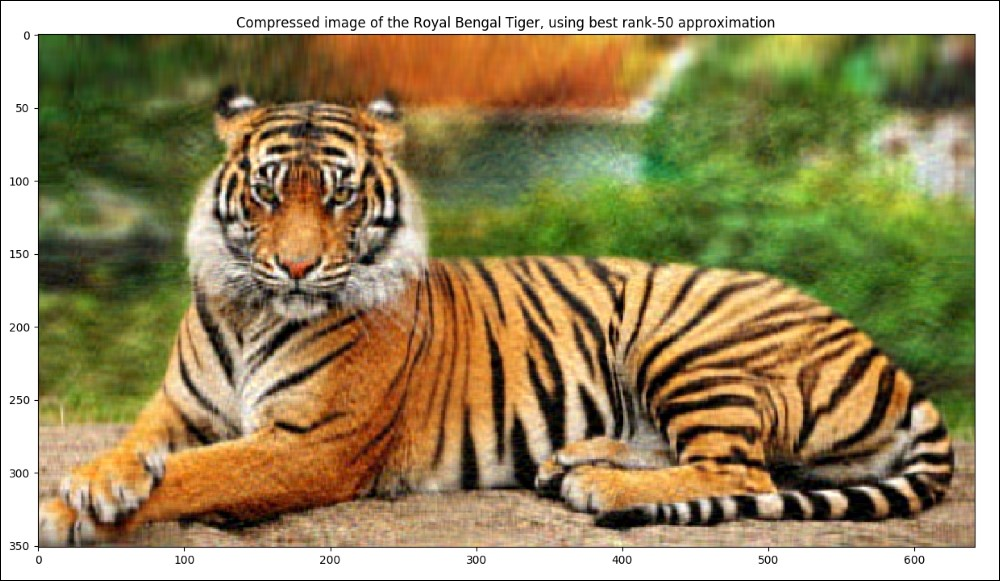Figure 22: The compressed one after best rank 10 approximations has only 610KB in size with lower resolution

However, using the best rank 50 approximations, we have observed that the compressed matrices have a total size of about 3048 KB, which is about 12 times less. Let's see the compressed one: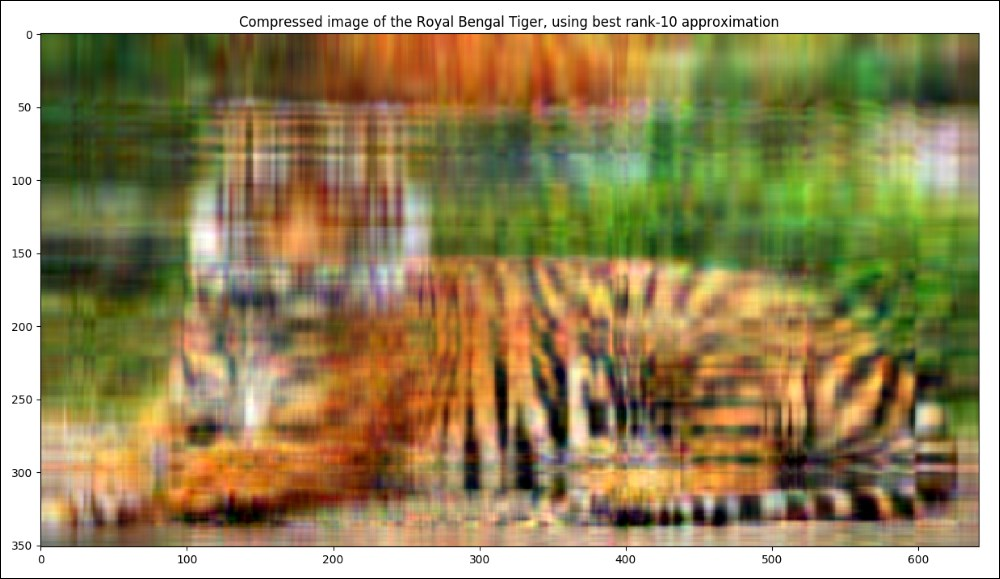Figure 23: The compressed one after best rank 50 approximations has only 3048 KB in size with better resolution

Using the best rank 200 approximations, the size of the compressed one is: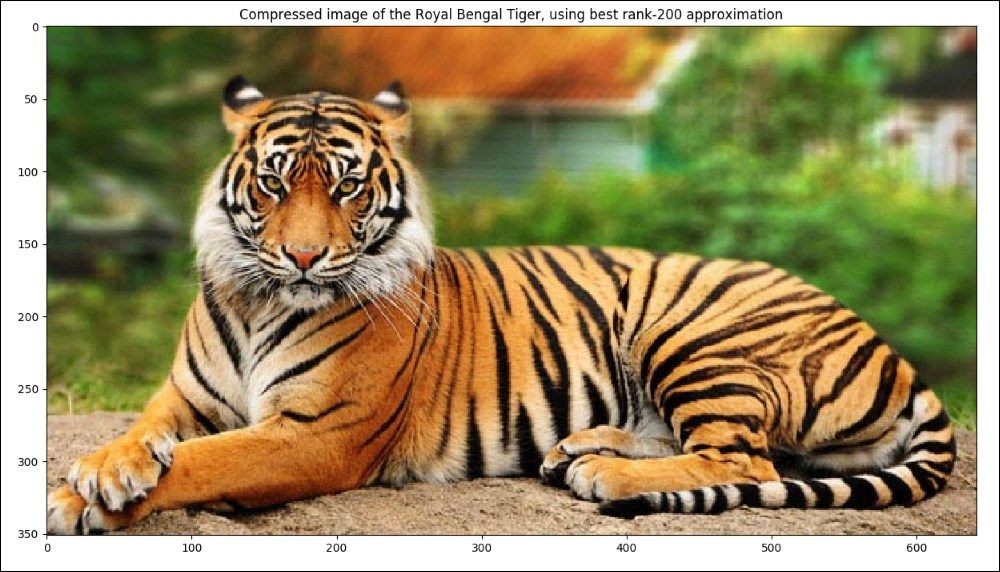Figure 24: The compressed one after best rank 200 approximations with full resolution

Now the preceding images of the tiger can be generated using SVD. Just execute Python3 `SVD_Demo.py`.

## Predictive analytics tools in Python

We will see throughout this book that Python is a great tool for developing predictive models that can be used for predictive analytics. There are many other tools and frameworks have been developed around it such as TensorFlow, H20, Caffe, Theano, PyTorch, and so on.

TensorFlow is mathematical software and an open-source software library for machine intelligence, developed in 2011, by the Google brain team. The initial target of TensorFlow was to conduct research in machine learning and in deep neural networks. However, the system is general enough to be applicable in a wide variety of other domains such as numerical computation using data flow graphs that enables machine learning practitioners to do more data-intensive computing. It provides some robust implementation of the widely used implementation of deep learning algorithms. TensorFlow offers you a very flexible architecture that enables you to deploy computation to one or more CPUs or GPUs in a desktop, server or mobile device with a single API.

Theano is probably the most widespread library. Written in Python, one of the languages most used in the field of machine learning (also in TensorFlow), allows the calculation using the GPU, which has 24x performance, even better than the CPU. It allows you to define, optimize, and efficiently evaluate complex mathematical expressions such as multidimensional arrays.

Other predictive analytics tools include Matlab, Torch, Weka, KNIME, SAS, SPSS, R, Mahout, Minitab, SAM, StatSoft, and so on.

Throughout this book, we will be using TensorFlow only. A more detail discussion is beyond the scope of this book. However, interested readers can read about and explore other tools and frameworks.

## Summary

Linear algebra plays an important role in predictive analytics especially in machine learning and also in broader mathematics. In this chapter, we have tried to provide a very basic introduction to predictive analytics. We have seen where and why to use this. Then we have seen how linear algebra helps in learning predictive modeling. We have seen how to perform SVD and PCA operations using Python modules. Finally, we have had a quick look at the widely used predictive analytics tools in Python.

In the next chapter, we will cover some statistical concepts before getting started with predictive analytics formally. For example, random sampling, hypothesis testing, chi-square test, correlation, expectation, variance, covariance and Bayes' rule, and so on. In the second part of this chapter, we will discuss probability and information theory for the predictive analytics.

Information theory that deals with the quantification, storage, and communication of information will be discussed too. Probability theory, which is a branch of mathematics concerned with probability, and the analysis of random phenomena will be discussed in the last section to help the data scientist gain more insight while performing predictive analytics.